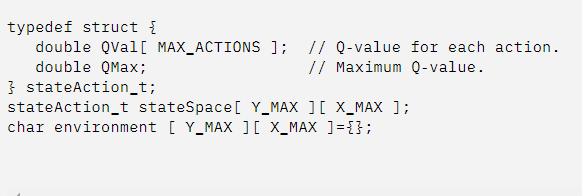# Give example of sample implementation of Q-learning

In this modest approach, I establish a 20x20 cell environment.
An obstacle (such as a wall or impassible object), open space (with a reward value of 0), and the goal cell can all be found in each cell (with a reward value of 1).

The agent starts at the upper-left cell and utilizes Q-learning to determine the best path to the goal, displayed after the learning process.

Let’s start with the fundamental structures that I used to implement Q-learning. The state–action structure is the first, and it contains the Q-values for a state’s actions.

Within any given state, the MAX ACTIONS symbol symbolises the four actions that an agent can execute (0 = North, 1 = East, 2 = South, 3 = West).

Val represents the Q-values for each of the four actions, and the maximum Q-value is represented by QMax (used to determine the best path when the agent uses its learned knowledge).

For each cell in the environment, this structure is created.
Characters (|, +, -, # as barriers;" as open space; and \$ as the desired state) are also used to build an environment:Let’s start with the essential function. This function implements the Q-learning loop, in which the agent takes actions at random, updates the Q-values, and displays the optimal path to the objective after a certain number of epochs:

The ChooseAgentAction function then determines the agent’s following action depending on the desired policy (explore versus exploit).
The function identifies and returns the action with the highest Q-value for the exploit policy.

This option is the optimal course of action for this situation, and it reflects the agent’s use of knowledge to move fast toward the goal.

I get a random action for the explore policy and then return it if it’s a good action (moves the agent to the goal or open space and not into an obstacle).

Another option is to choose the action probabilistically as a function of QVal (using QVal as a probability over the sum of Q-values for the state):

In this simulation, the last function I’ll look at is UpdateAgent, which implements the Q-learning algorithm’s core.

It’s worth noting that this function comes with the desired action (a movement direction), which I use to determine the next stage to enter.
The reward from this state is taken from the environment, and the revised Q-value from the current state is calculated (and cache the QMax value, in case it has changed).

If the new state entered is the goal state, I reset the learning process by returning the agent to the original state:

For this demonstration, this short bit of code (along with a few others that you may look at on GitHub) implements Q-learning.

Make may be used to create the code, and then qlearn can run it.
The output of the code and the path selected as. Characters are shown in the diagram below.

This was done in the siimiliarity of the ExecuteAgent function (not shown), which uses the exploit policy to determine which path is best: